### Home > CALC > Chapter 8 > Lesson 8.4.1 > Problem8-144

8-144.
1.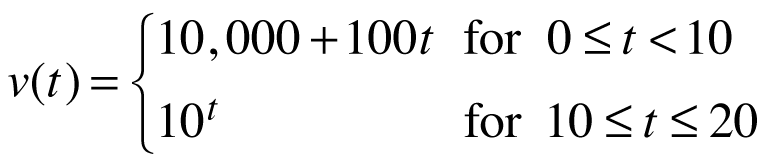The function v(t) below represents the velocity in kilometers per hour of the Starship Energize over a 20 second period during which the warp-drive was engaged. Homework Help ✎

2.1. What was the average velocity of the starship during this interval?

2. At what time, if any, was the ship's velocity equal to this average velocity? Does the Mean Value Theorem apply in this case? Explain.

3. What was the average acceleration of the starship during this interval?

4. At what time, if any, was the ship's acceleration equal to this average acceleration? Does the Mean Value Theorem apply in this case? Explain.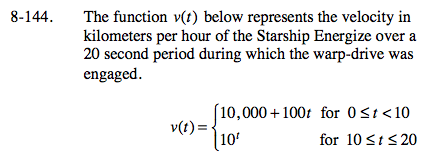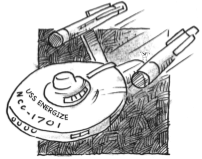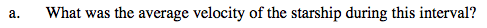You were given a velocity function. How will you find distance information?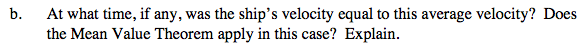MVT states that if a function is both continuous and differentiable on a closed interval, then the average value will equal the actual value somewhere.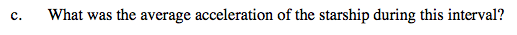Compare and contrast parts (a) and (c). Both are looking for averages. But, based on the function you were given, strategies to find averages are different.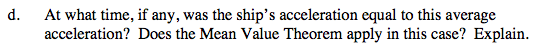MVT also states that if a function is both continuous and differentiable on a closed interval, then its average rate of change (AROC) will equal its actual rate of change (IROC) somewhere.